« Example 4.2.2-2 Unfavourable loadings and envelopes of stresses - deflections » Example 4.2.2-3 (project B_422-3) The structure of this example derives from the 1st example by removing beams b1, b2 and appending a cantilever at the side of beams b5 and b6.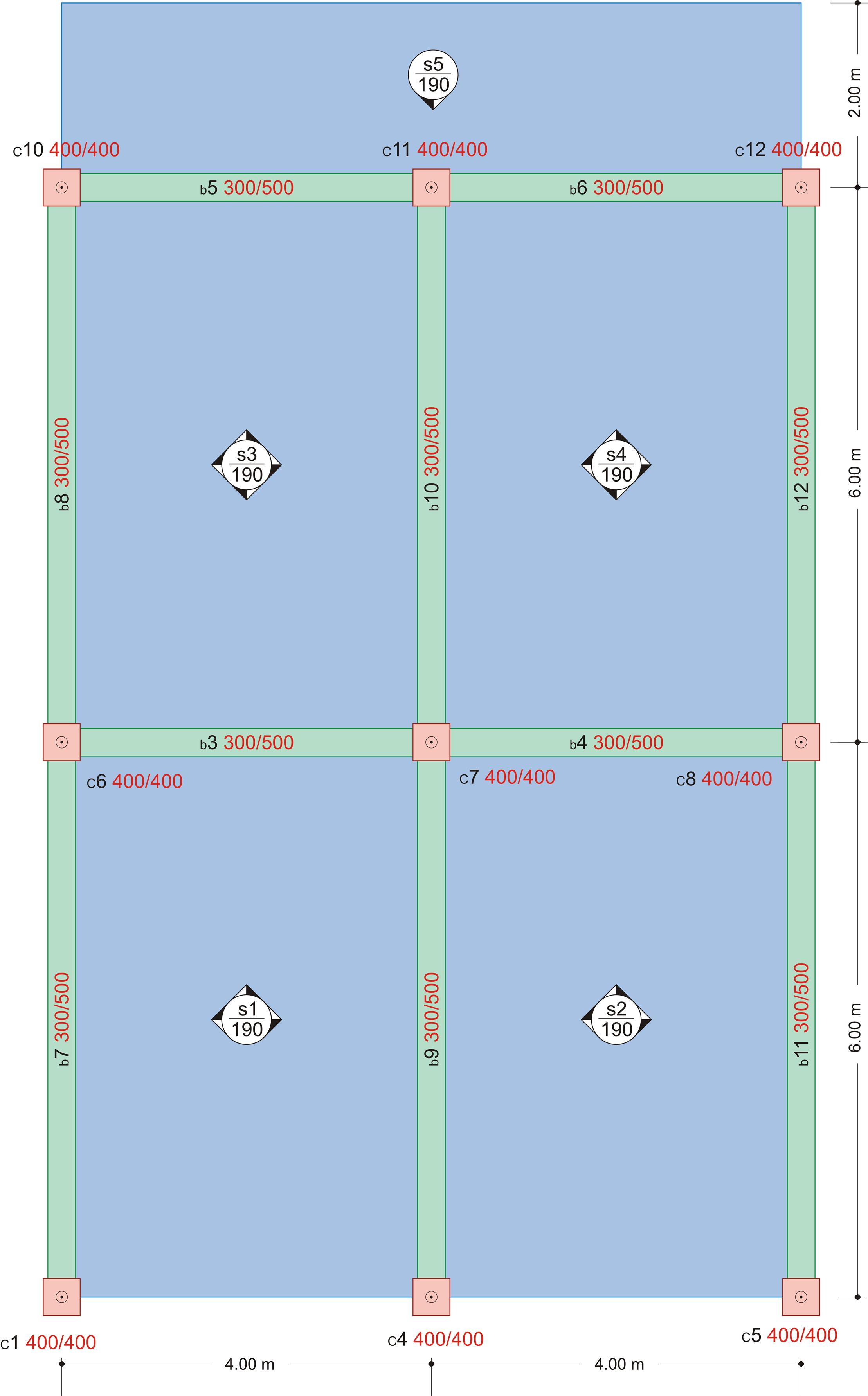Figure 4.2.2-41 Figure 4.2.2-41 The thickness of all slabs is 190 mm satisfying the slenderness requirements (see Volume C', chapter 6). S1, S2 are three-edge-supported slabs. Slab s5 is cantilever, carrying additional concentrated linear dead load G=1.0 kN/m on its free edges. The cantilever is fixed along the small side of the two-way slabs s3 and s4. To perform analysis set these parameters: Tab "Meshing": "Overall size" = 0.10 m, "Perimeter size" = 0.05 m, Tab "Modules": "SLABS" = ON, Tab "Loads": "Adverse Slabs" = ON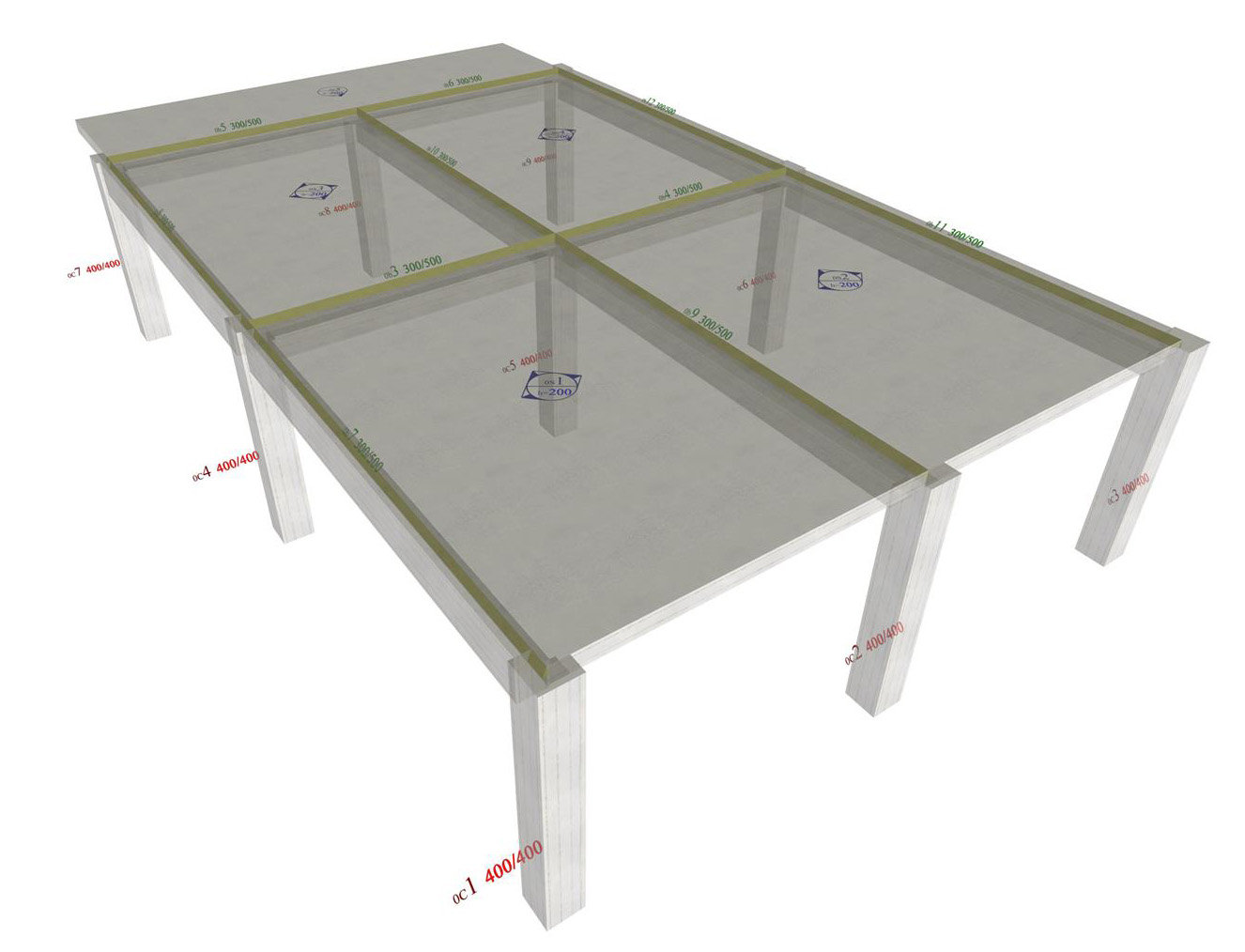Figure 4.2.2-42: The real stucture in 3D. Figure 4.2.2-42: The real stucture in 3D.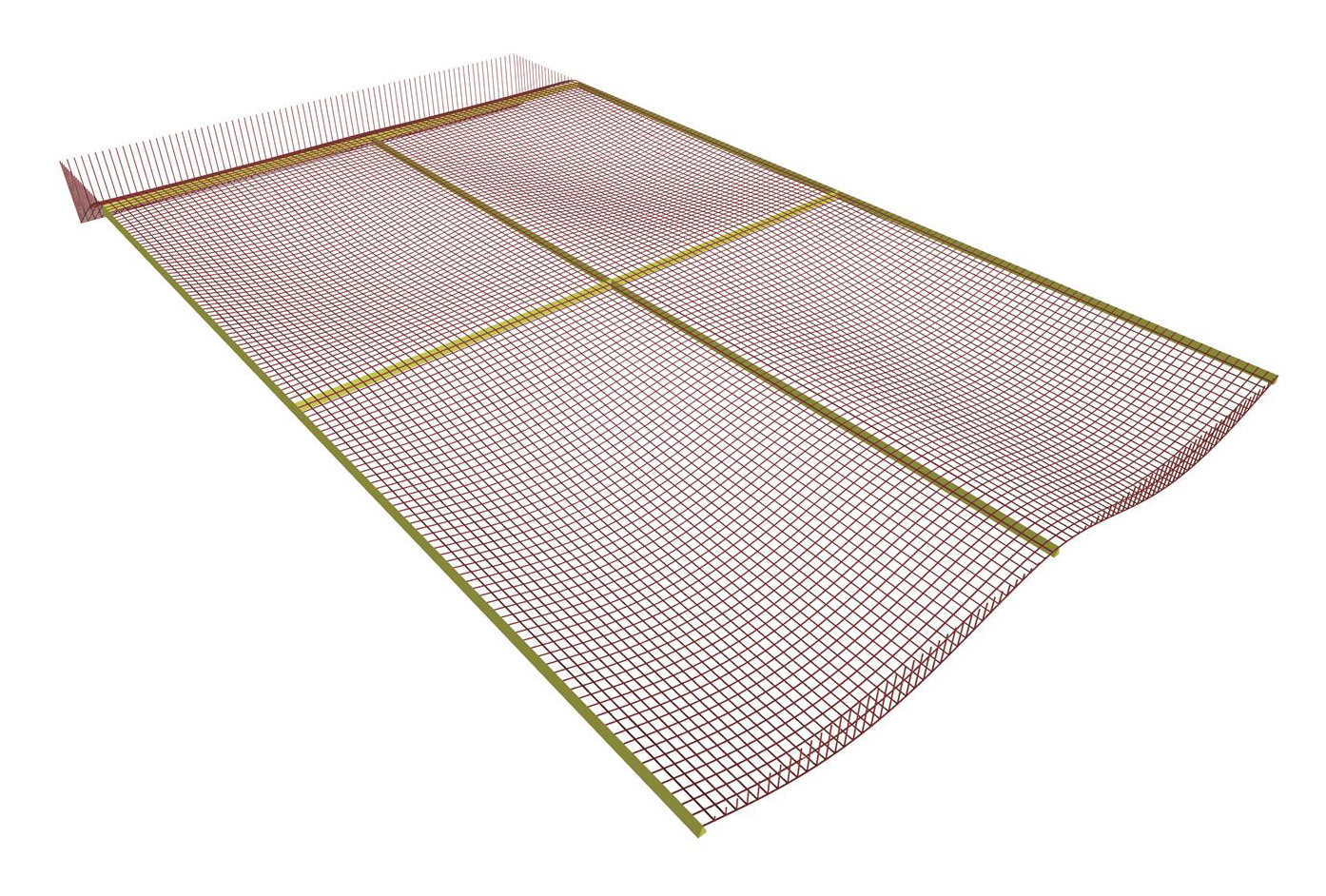Figure 4.2.2-43: The deformation of slabs in 3D. Figure 4.2.2-43: The deformation of slabs in 3D. Two, statically interesting, regions will be examined separately. The first comprises the two three-edge-supported slabs. The second comprises the cantilever and the two two-way slabs. The appropriate slabs are selected by pressing "Single" in the section "selection". Region of three-edge-supported slabs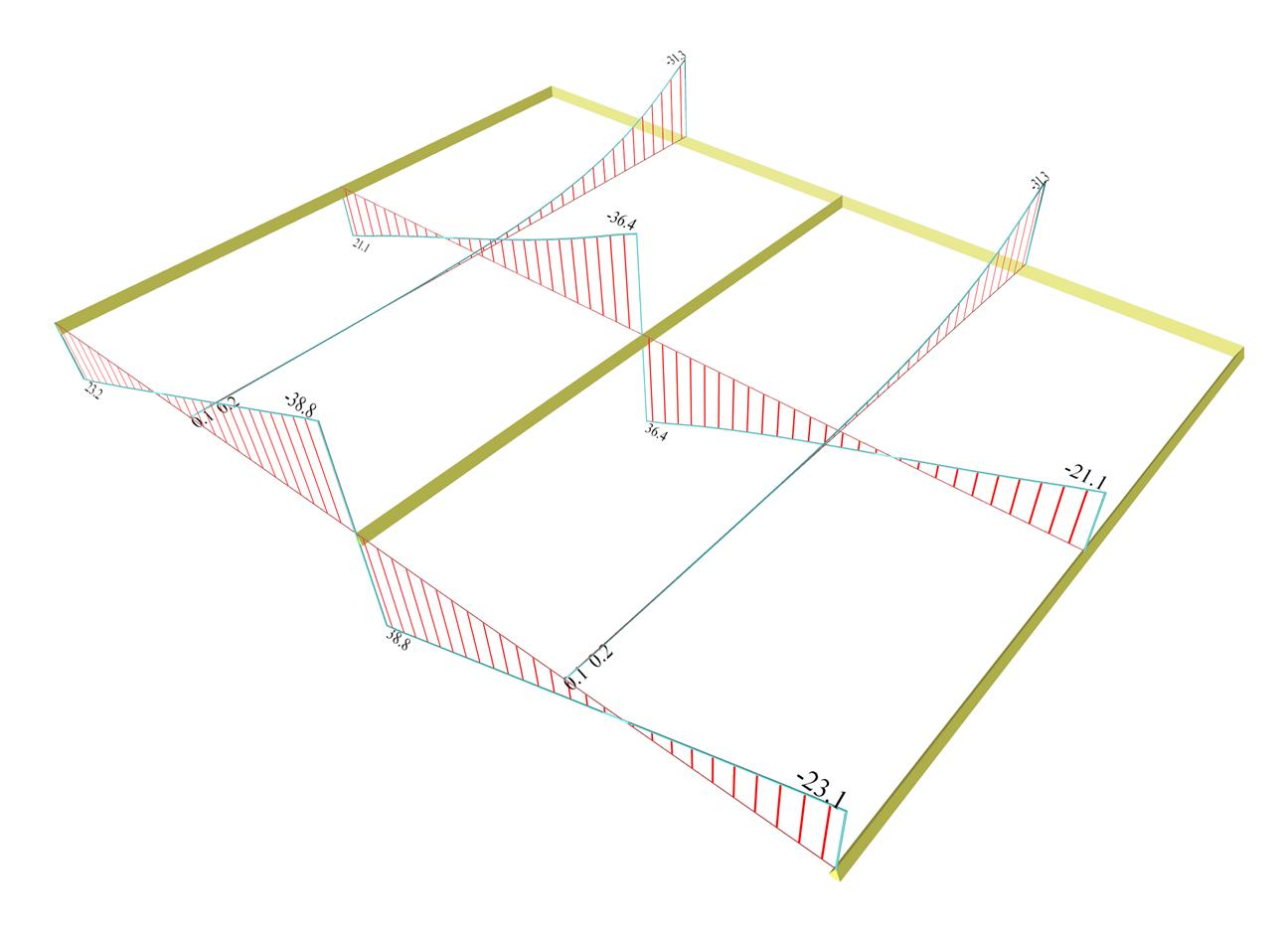Figure 4.2.2-44: Shear forces from Slab Results. Figure 4.2.2-44: Shear forces from Slab Results. The highest shears Vx varying from 23.1 to 38.8 kN, are developed at the free edge. Vx are somewhat smaller at the middle cross-section, varying from 21.1 to 36.4 kN. Shears Vy vary from 0.0 to 31.3 kN. The above values refer to the section of the Slab Results calculated from the integration of the stress resultants in distribution width 1.0 m. The respective values of the FEM Results Vx vary from 27.7 to 40.8 kN at the free edges and from 21.1 to 36.6 kN at the middle cross-section. Shears Vy from 0.0 to 31.9 kN. These differences are considerable only in the region of the free edges where there is a "crumple" as shown in the following 3D distribution of shears and especially in the side view of the 3D.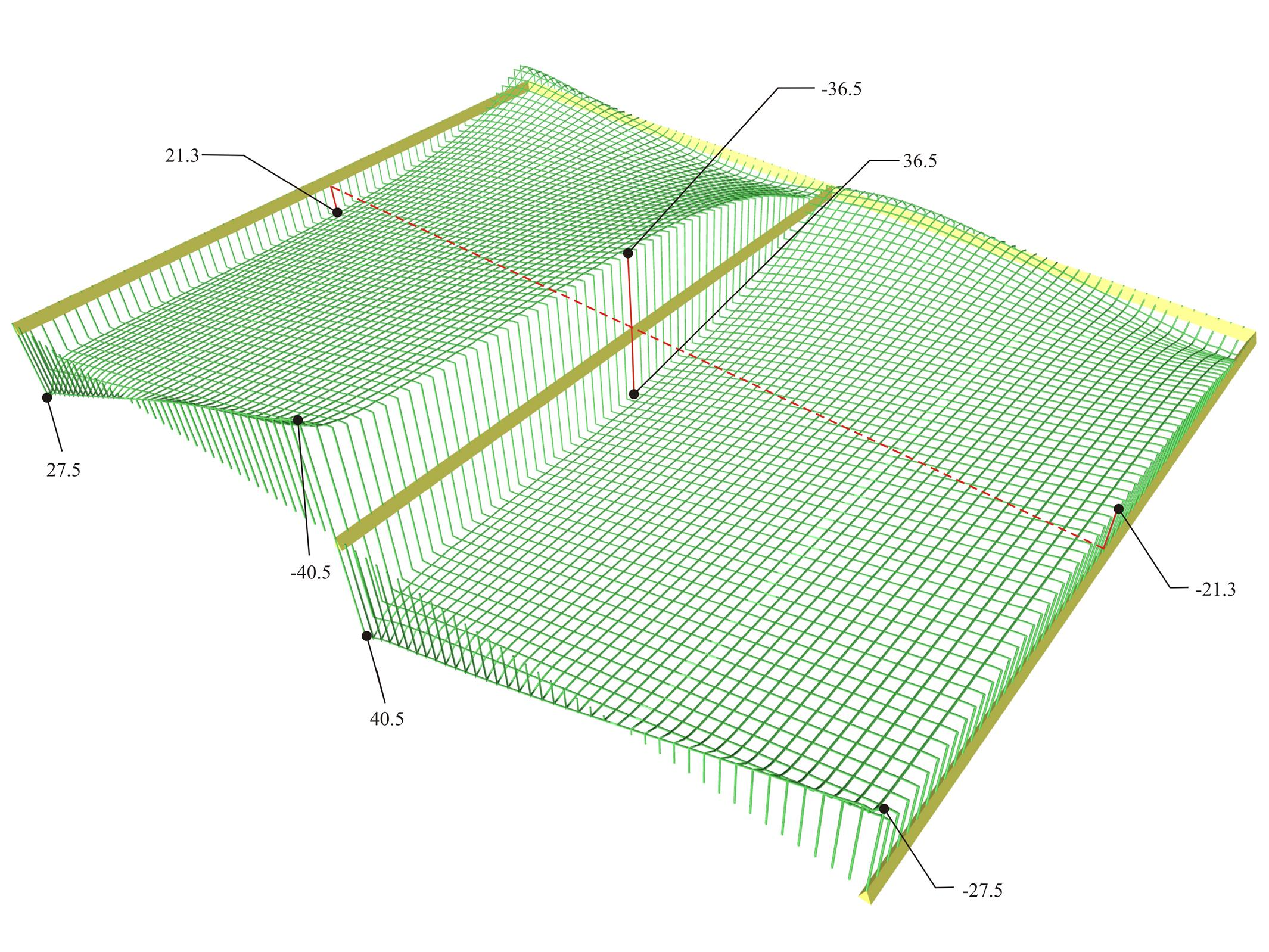Figure 4.2.2-45: Distribution of shear forces [Vx] ([V11] in FEM Results, detailed per 0.10 m) in 3D. Figure 4.2.2-45: Distribution of shear forces [Vx] ([V11] in FEM Results, detailed per 0.10 m) in 3D.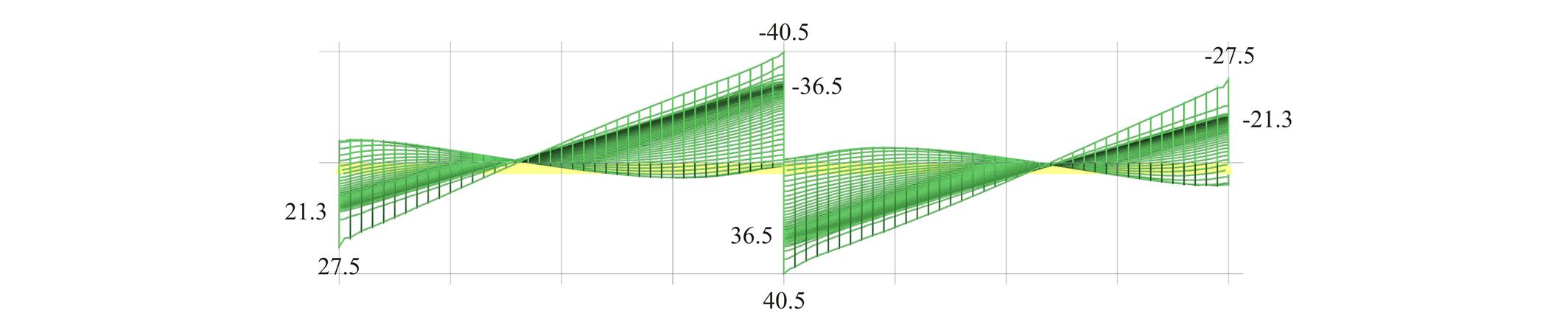Figure 4.2.2-46: Front view of the 3D distribution of shear forces [Vx] ([V11] in FEM Results). Figure 4.2.2-46: Front view of the 3D distribution of shear forces [Vx] ([V11] in FEM Results).Figure 4.2.2-47: Side view of the 3D distribution of shear forces [Vx] ([V11] in FEM Results). Figure 4.2.2-47: Side view of the 3D distribution of shear forces [Vx] ([V11] in FEM Results).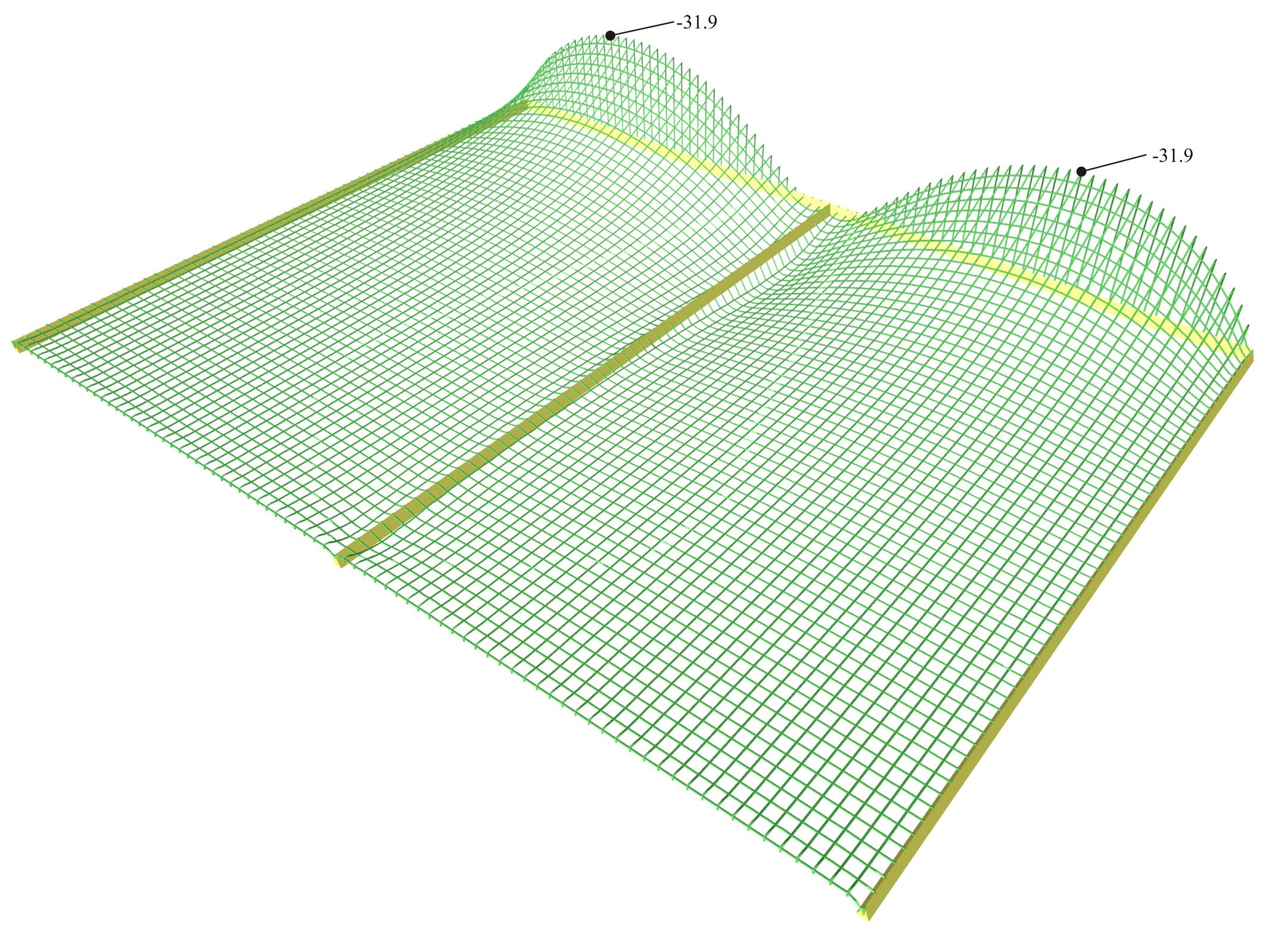Figure 4.2.2-48: Shear forces [Vy] ([V22] in FEM Results, details per 0.1 m) extend only in the regions of the middle supports. Figure 4.2.2-48: Shear forces [Vy] ([V22] in FEM Results, details per 0.1 m) extend only in the regions of the middle supports.Figure 4.2.2-49: Front view of the 3D distribution of shear forces [Vy] ([V22] in FEM Results). Figure 4.2.2-49: Front view of the 3D distribution of shear forces [Vy] ([V22] in FEM Results).Figure 4.2.2-50: Side view of the 3D distribution of shear forces [Vy] ([V22] in FEM Results). Figure 4.2.2-50: Side view of the 3D distribution of shear forces [Vy] ([V22] in FEM Results).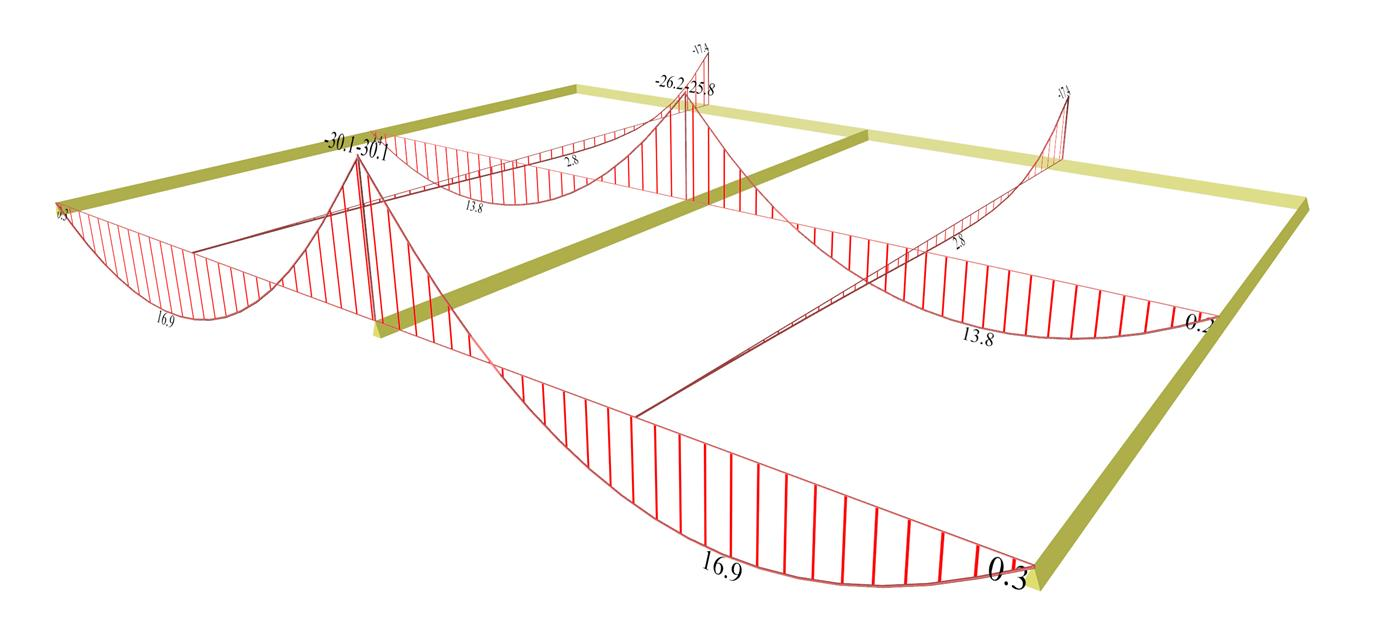Figure 4.2.2-51: Bending moments from Slab Results. Figure 4.2.2-51: Bending moments from Slab Results. One notices that the higher moments Mx, 16.9 kNm at the opening and -30.0 kNm at the support, are developed at the free edge. The values of Mx are lower at the middle cross-section, (13.8 kNm at the opening and -26.0 kNm at the support). Moments My vary from 0.0 to -17.4 kN. These values refer to the area of the Slab Results calculated from the integration of the stress resultants in distribution width of 1.0 m. The respective values of the FEM Results for Mx are: at free edges, 17.0 at the opening and -30.5 kNm at the support, whereas at the middle cross-section, 13.9 at the opening and -26.2 kNm at the support. For moments My, in the middle cross-section values vary from 0.0 to -18.2 kNm. The differences are insignificant.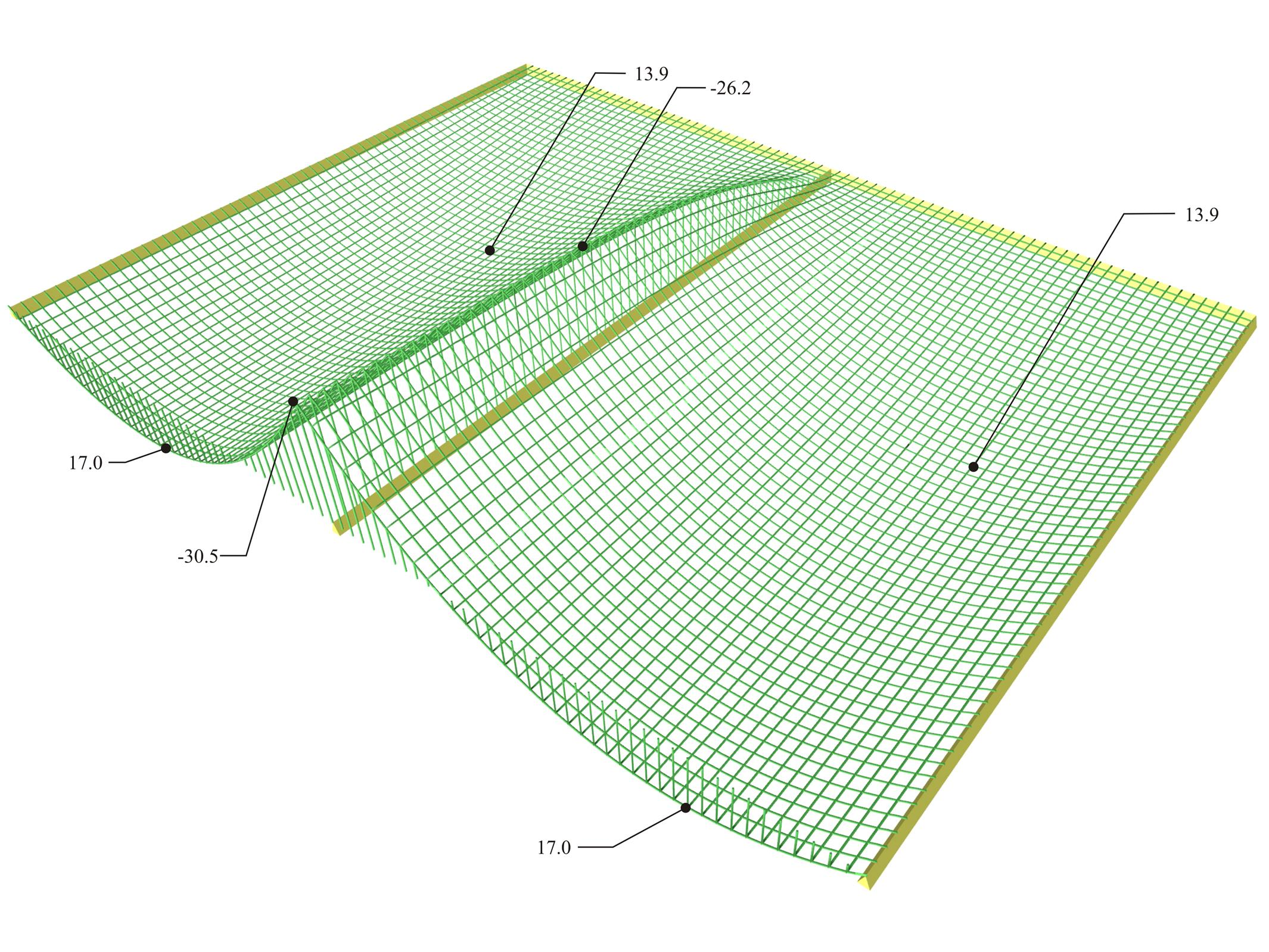Figure 4.2.2-52: Distribution of bending moments [Mx] ([M11] in FEM Results, details per 0.10 m) in 3D. Figure 4.2.2-52: Distribution of bending moments [Mx] ([M11] in FEM Results, details per 0.10 m) in 3D.Figure 4.2.2-53: Front view of the 3D distribution of bending moments [Mx] ([M11] in FEM Results). Figure 4.2.2-53: Front view of the 3D distribution of bending moments [Mx] ([M11] in FEM Results).Figure 4.2.2-54: Side view of the 3D distribution of bending moments [Mx] ([M11] in FEM Results). Figure 4.2.2-54: Side view of the 3D distribution of bending moments [Mx] ([M11] in FEM Results).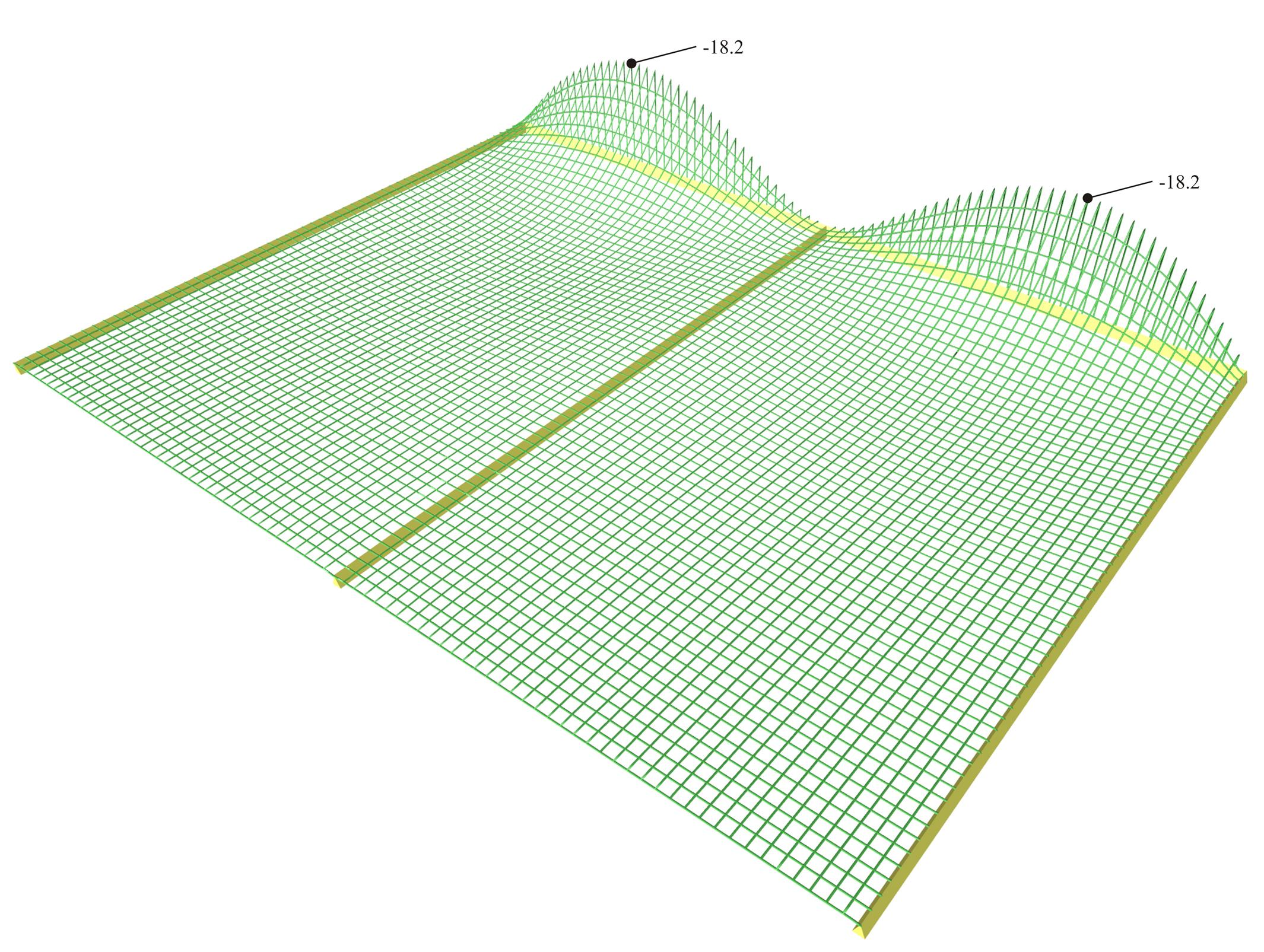Figure 4.2.2-55: Distribution of bending moments [My] ([M22] in FEM Results, details per 0.1 m) extend only in the regions of the top supports. Figure 4.2.2-55: Distribution of bending moments [My] ([M22] in FEM Results, details per 0.1 m) extend only in the regions of the top supports.Figure 4.2.2-56: Front view of the 3D distribution of bending moments [My] ([M22] in FEM Results). Figure 4.2.2-56: Front view of the 3D distribution of bending moments [My] ([M22] in FEM Results).Figure 4.2.2-57: Side view of the 3D distribution of bending moments [My] ([M22] in FEM Results). Figure 4.2.2-57: Side view of the 3D distribution of bending moments [My] ([M22] in FEM Results).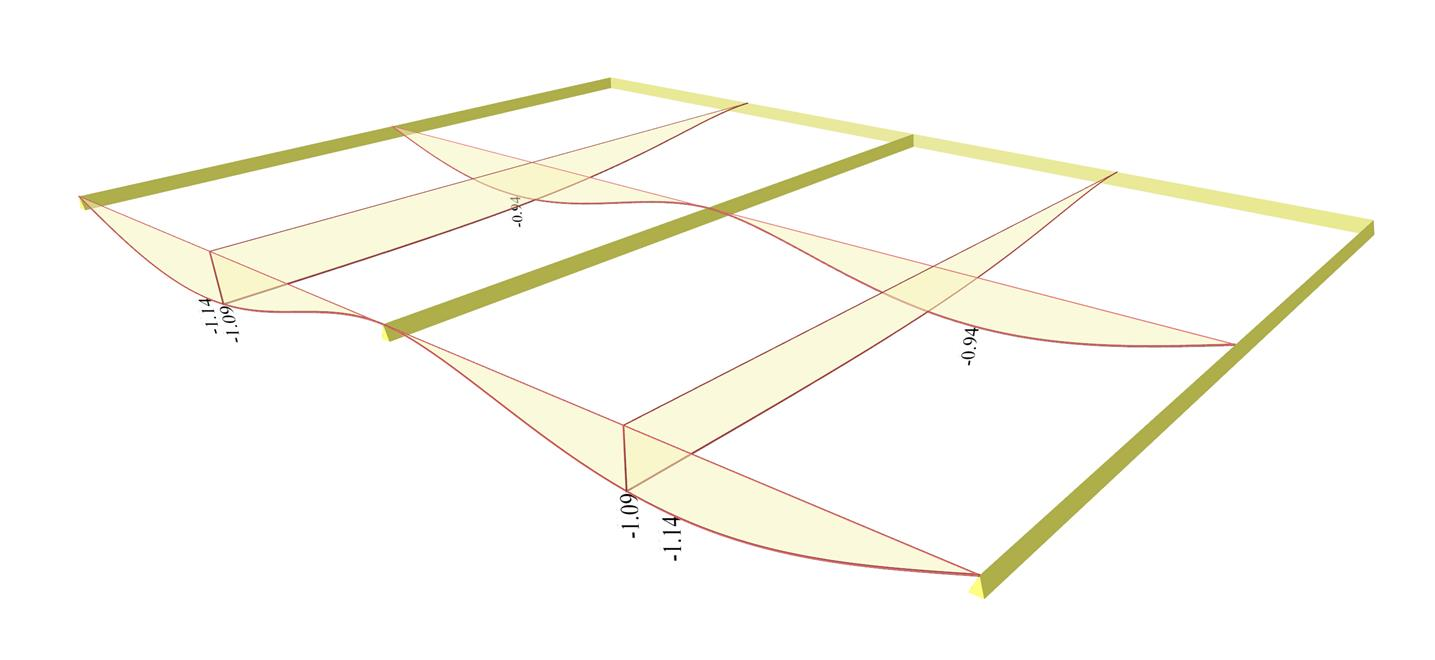Figure 4.2.2-58: Deflections from Slabs Results. Figure 4.2.2-58: Deflections from Slabs Results. The maximum deflection developed at the free edges is equal to -1.14 mm, whereas at the middle cross-sections is equal to -0.94 mm. Cantilever region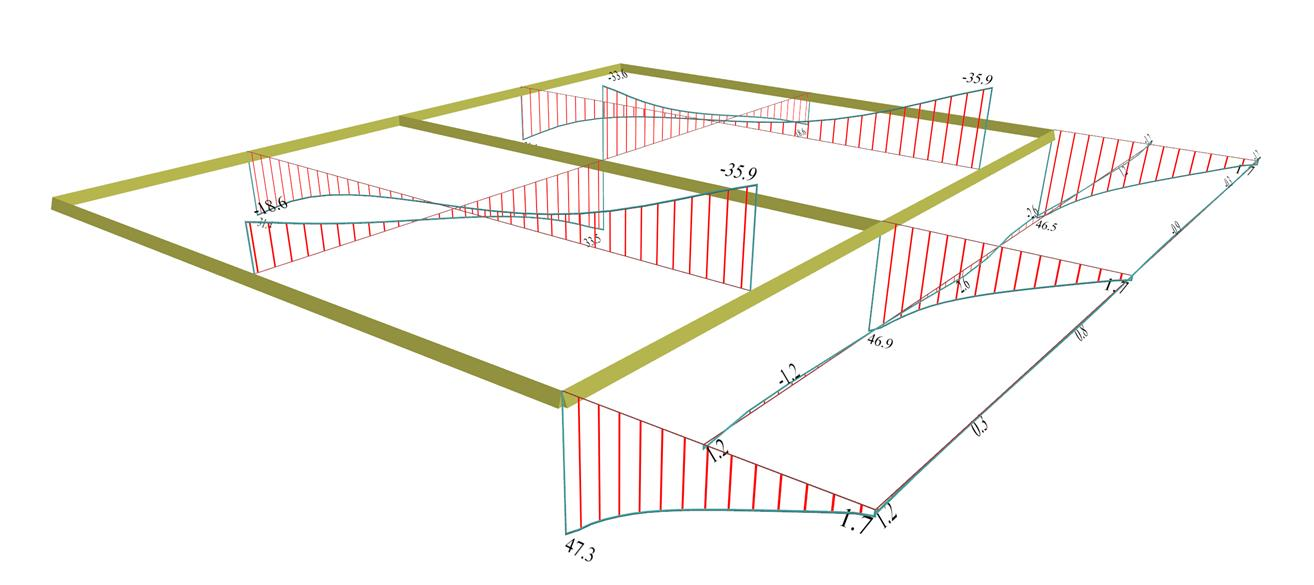Figure 4.2.2-59: Shear forces from Slab Results. Figure 4.2.2-59: Shear forces from Slab Results. Shears Vx in the middle cross-sections of the two two-way slabs, vary from 18.6 to 33.6 kN compared to the almost same values 18.8 to 33.3 of the 1st example. This means that the effect of the adjacent three-edge-supported slabs and the effect of the cantilever are insignificant at the middle region of the slab along x. The same applies to the shears on the supports to the three-edge-supported slabs where their value 31.4 kN differ insignificantly from the respective shears of the 1st example. At the cantilever regions close to the three transverse linear supports of the two adjacent two-way slabs, shear forces are high due to concentration of extra loads transferred from the immediately adjacent slabs. This specific influence is well shown in the following figures, containing the detailed shear forces and bending moments distributions.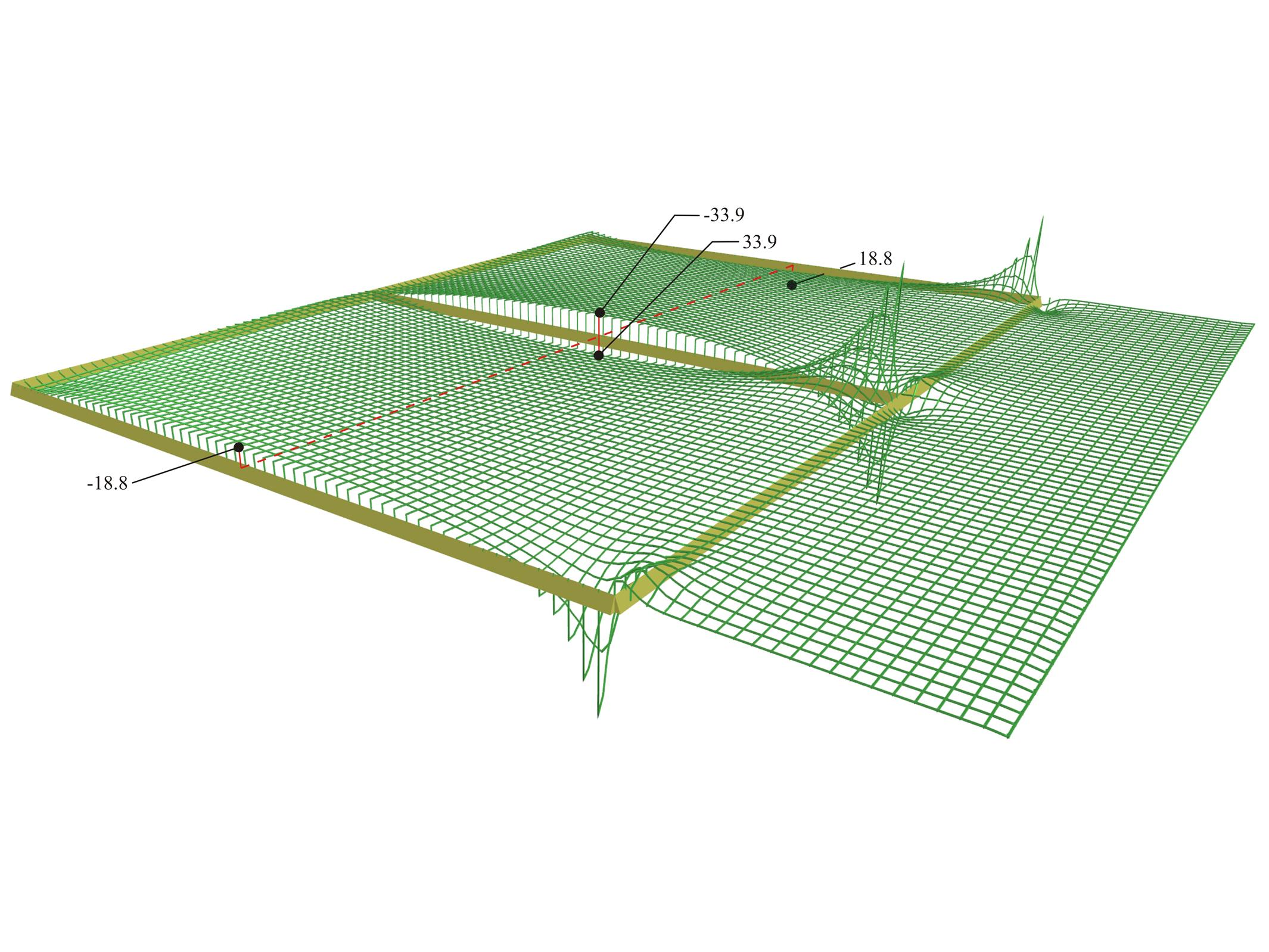Figure 4.2.2-60: Distribution of shear forces [Vx] (V11 from FEM Results, details per 0.10 m) in 3D. Figure 4.2.2-60: Distribution of shear forces [Vx] (V11 from FEM Results, details per 0.10 m) in 3D.Figure 4.2.2-61: Front view of the 3D distribution of shear forces [Vx] ([V11] in FEM Results). Figure 4.2.2-61: Front view of the 3D distribution of shear forces [Vx] ([V11] in FEM Results).Figure 4.2.2-62: Side view of the 3D distribution of shear forces [Vx] ([V11] in FEM Results). Figure 4.2.2-62: Side view of the 3D distribution of shear forces [Vx] ([V11] in FEM Results). The extremely high shears in the region of the two-way slabs behind the cantilever is due to the negative load developed directly behind the cantilever in order to balance its strong moment My.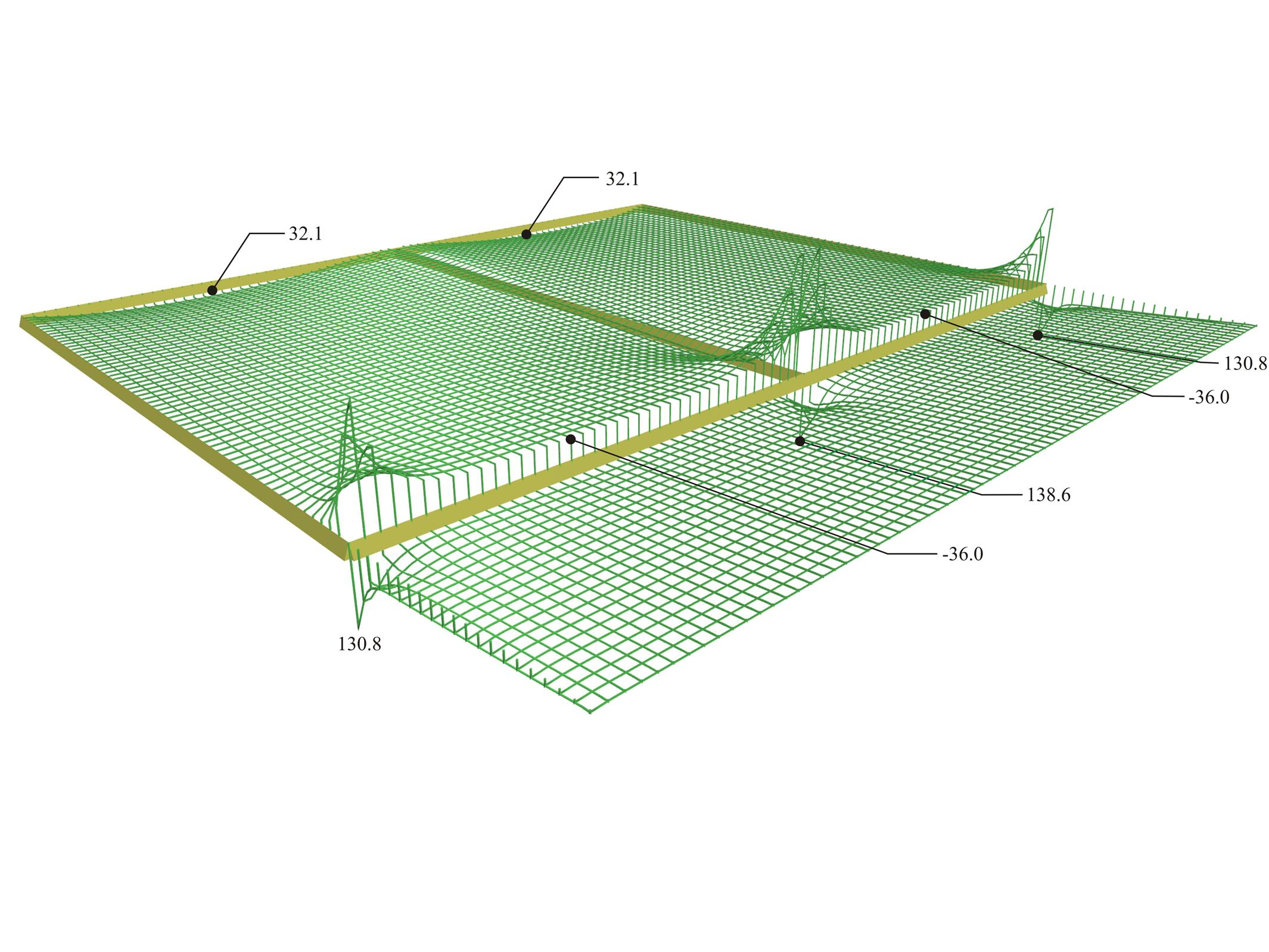Figure 4.2.2-63: Shear forces [Vy] ([V22] in FEM Results, detailed per 0.1 m) extend only in the regions of middle supports. Figure 4.2.2-63: Shear forces [Vy] ([V22] in FEM Results, detailed per 0.1 m) extend only in the regions of middle supports.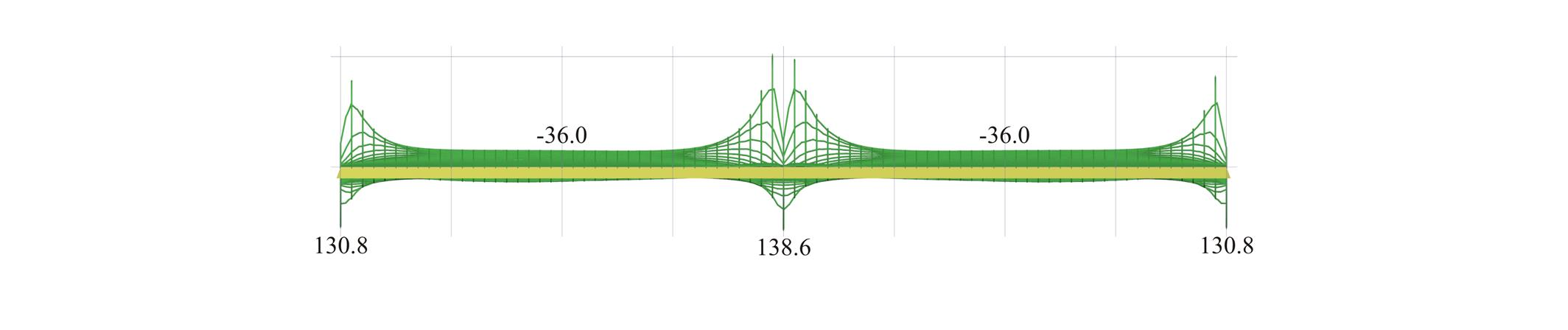Figure 4.2.2-64: Front view of the 3D distribution of shear forces [Vy] ([V22] in FEM Results). Figure 4.2.2-64: Front view of the 3D distribution of shear forces [Vy] ([V22] in FEM Results).Figure 4.2.2-65: Side view of the 3D distribution of shear forces [Vy] ([V22] in FEM Results). Figure 4.2.2-65: Side view of the 3D distribution of shear forces [Vy] ([V22] in FEM Results). At the points of the slab (in this case of the cantilever) where fixed transverse support exist, numerous peaks are created, mainly for shears (in this case 130.8 and 138.6 kN) and secondarily for moments. These regions are forced to carry large part of the load of the adjacent slabs mainly near the supports. This is the reason why the occurrence of high shears shortly before and shortly after the support. However, these shears are taken into account in detail by considering their average values in a width, e.g. 1.0 m, which equals to 47.0 kN (Slab Results).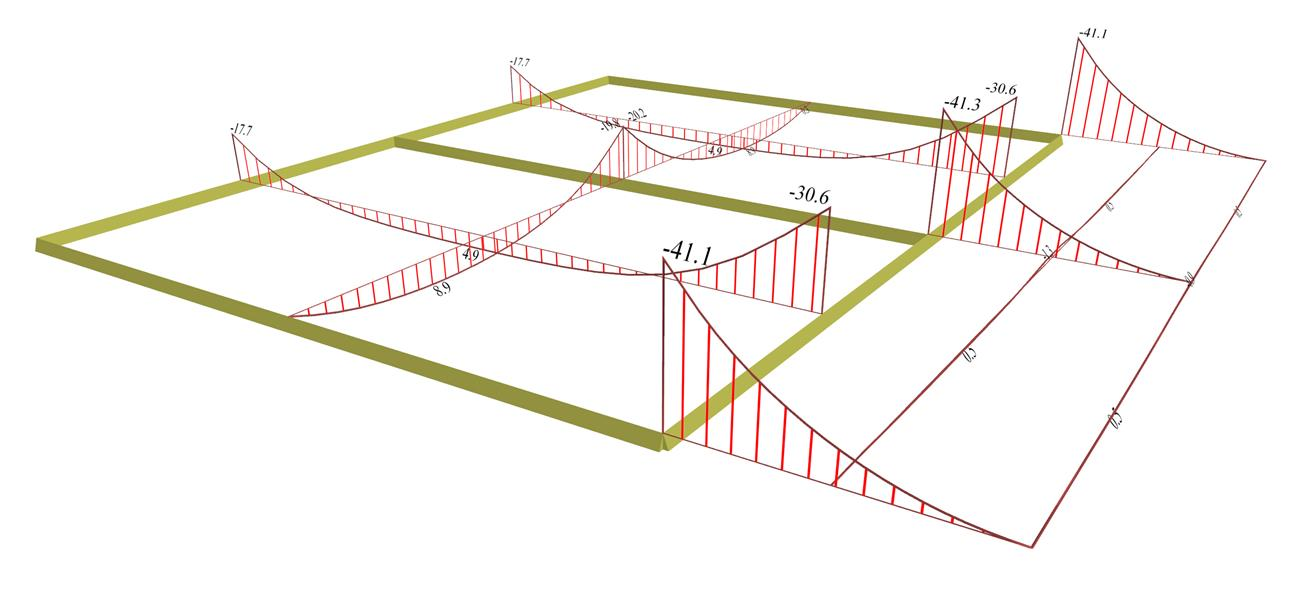Figure 4.2.2-66: Bending moments from Slab Results. Figure 4.2.2-66: Bending moments from Slab Results. Two-way slabs' span moments, are relatively low (Mx=8.9 kNm and My=4.9 kNm), compared to the respective values of the 1st example (10.8 and 4.1 kNm). At the common support, Mx,erm=-20.0 kNm (the respective value of the 1st example: -22.0 kNm), whereas at the border with the three-edge-supported slabs is My,erm=-17.4 kNm (the respective value of the 1st example: -16.2 kNm). It is concluded that the stress resultants on most of the area of the two-way slabs are similar to those of the 1st example. In the region of the continuity with the cantilever support moments are -30.6 kNm equal to almost isostatic moment of the cantilever. The higher moments of the cantilever -41.0 kNm are the local peak moments, as shown in the next figure in the detailed distribution of the My due to the fixed transverse supports.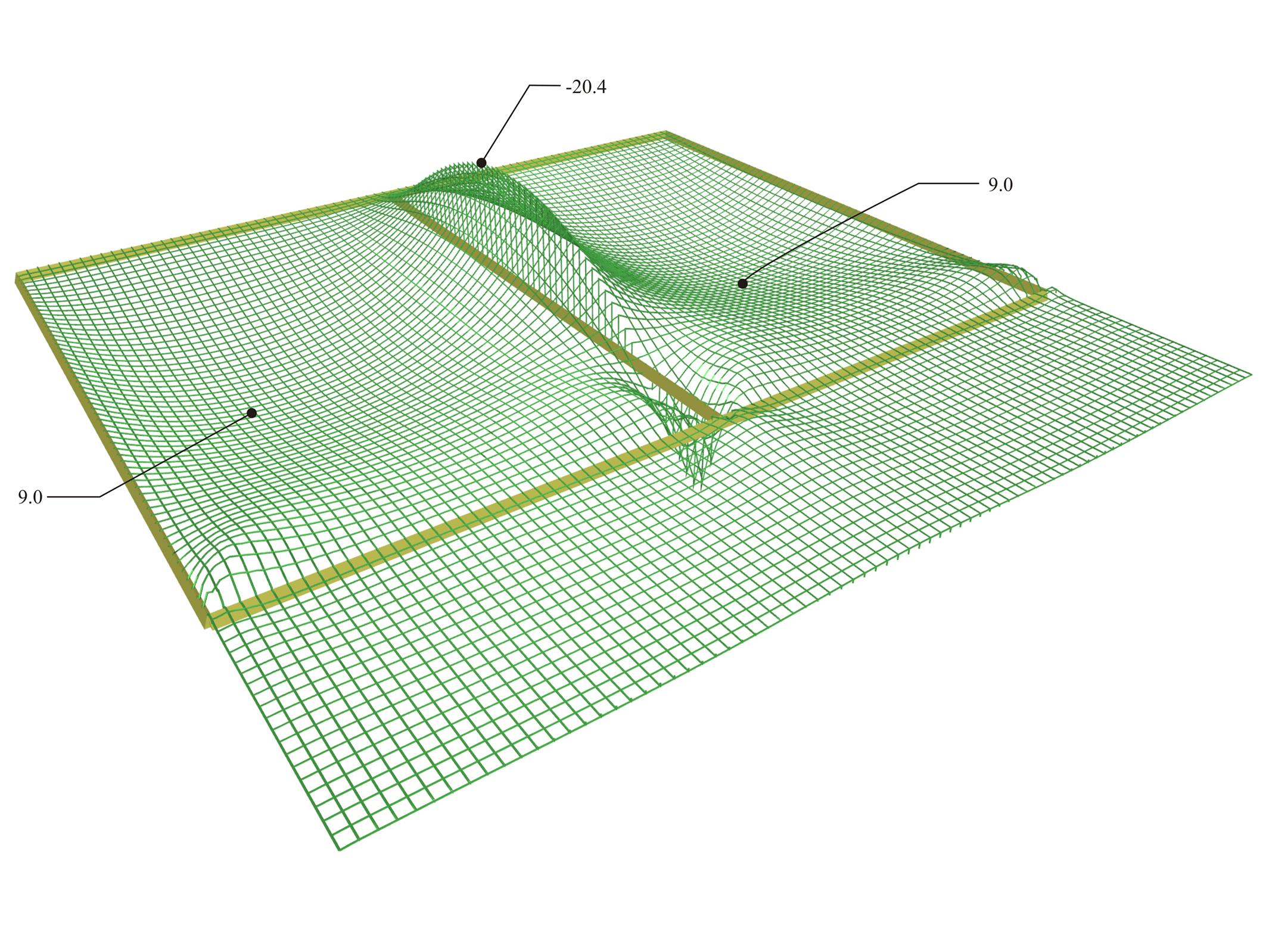Figure 4.2.2-67: Distribution of bending moments [Mx] ([M11] in FEM Results, detailed per 0.10 m) in 3D. Figure 4.2.2-67: Distribution of bending moments [Mx] ([M11] in FEM Results, detailed per 0.10 m) in 3D.Figure 4.2.2-68: View of the 3D distribution of bending moments [Mx] ([M11] in FEM Results). Figure 4.2.2-68: View of the 3D distribution of bending moments [Mx] ([M11] in FEM Results).Figure 4.2.2-69: Side view of the 3D distribution of bending moments [Mx] ([M11] in FEM Results). Figure 4.2.2-69: Side view of the 3D distribution of bending moments [Mx] ([M11] in FEM Results). The positive peak moments forming a sharp "hole", at the region of the support exactly behind the cantilever, results from the negative load created at this region by the cantilever's moment. Notice that the influence of the strong cantilever, both in negative and positive moments, decreases in a small distance from the cantilever support. The insignificant differences in values of moments Mx between FEM results and Slab results are due to the relatively small curvature of the 3D moment diagram Mx.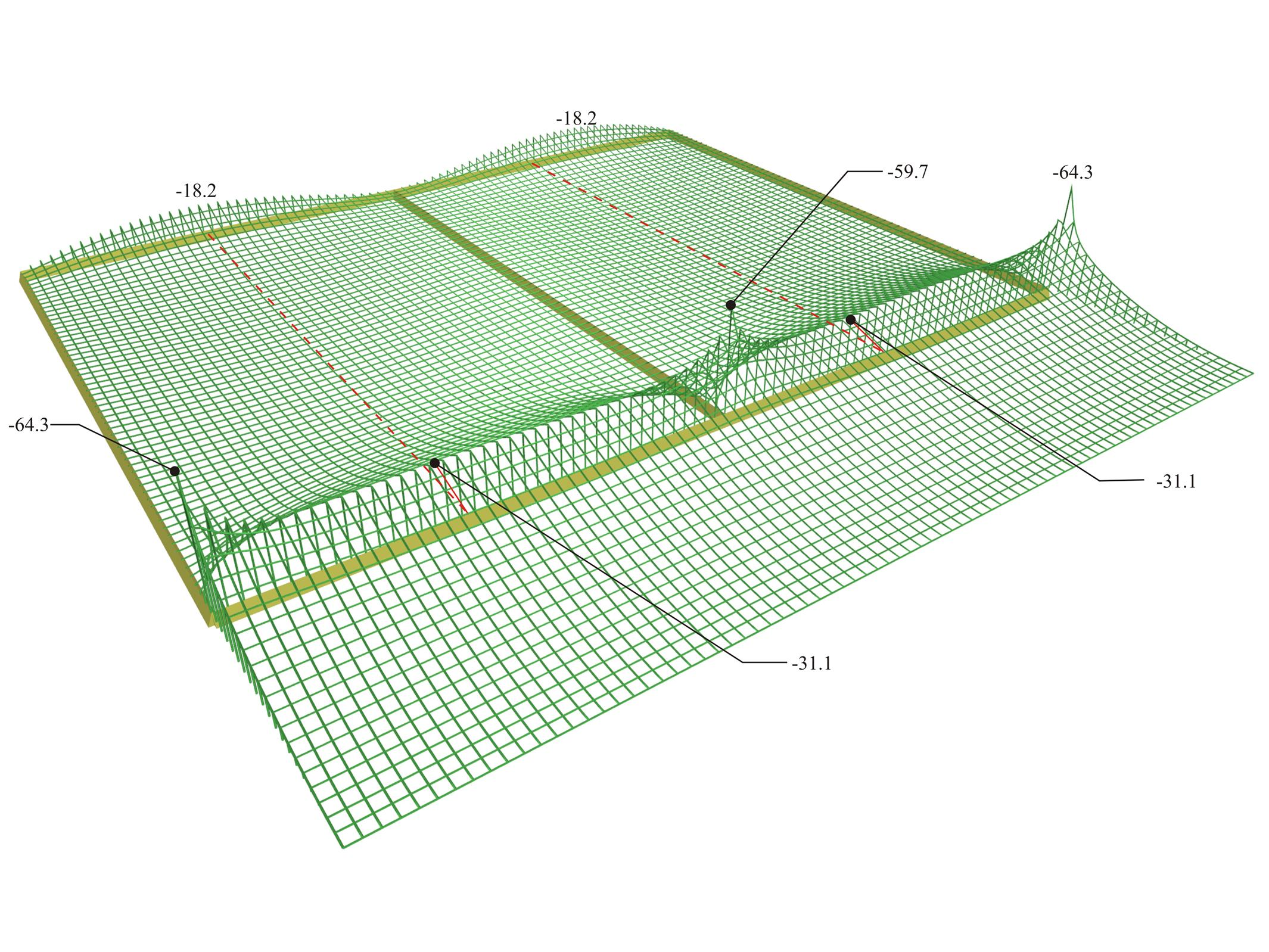Figure 4.2.2-70: Distribution of bending moments [My] ([M22] in FEM Results, detailed per 0.1 m) extend only in the regions of top supports. Figure 4.2.2-70: Distribution of bending moments [My] ([M22] in FEM Results, detailed per 0.1 m) extend only in the regions of top supports.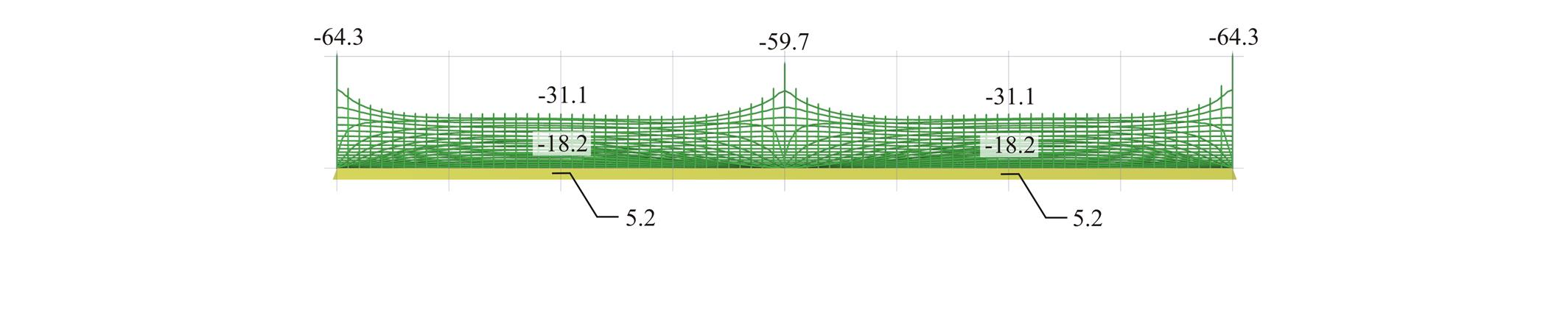Figure 4.2.2-71: Front view of the 3D distribution of bending moments [My] ([M22] in FEM Results). Figure 4.2.2-71: Front view of the 3D distribution of bending moments [My] ([M22] in FEM Results).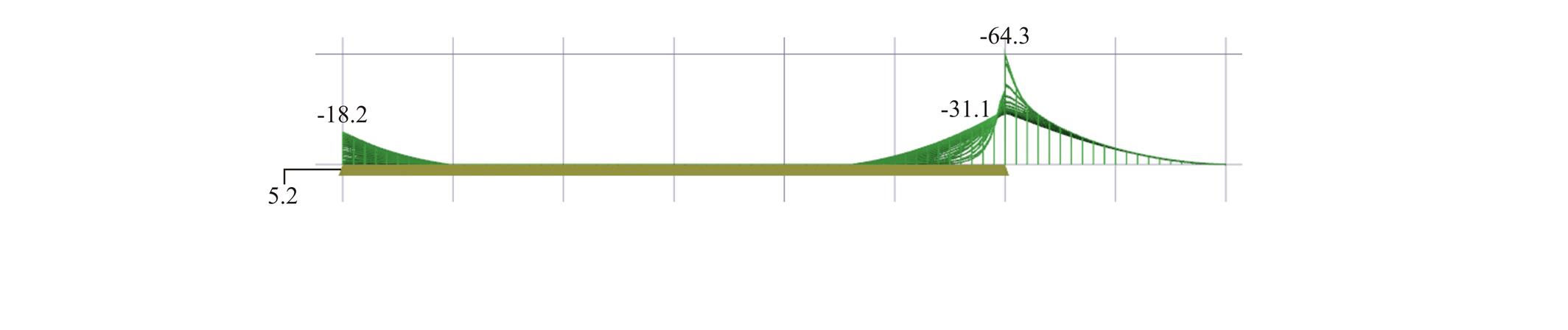Figure 4.2.2-72: Side view of the 3D distribution of bending moments [My] ([M22] in FEM Results). Figure 4.2.2-72: Side view of the 3D distribution of bending moments [My] ([M22] in FEM Results). Negative moments are developed on most of the region of the two-way slabs due to the strong cantilever support. Along most of the support of the cantilever and the two-way slabs, bending moments have a value of -31.1 kNm whereas near the supports peak values occur within a width less than 1.0 m.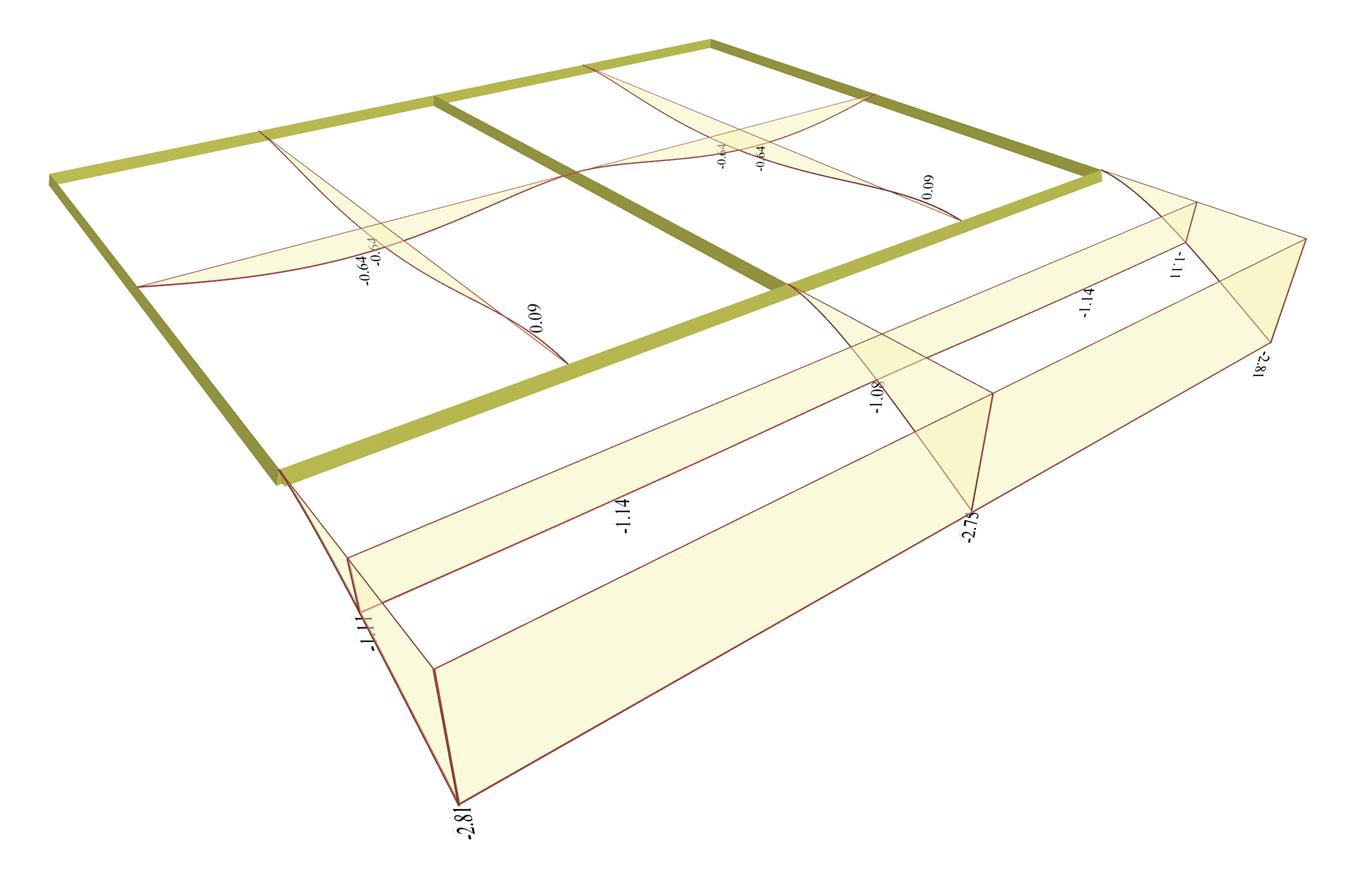Figure 4.2.2-73: Deflections from Slabs Results. Figure 4.2.2-73: Deflections from Slabs Results. The maximum elastic deflection along the cantilever free edge varies from -2.75 mm at the middle to -2.81 mm at both ends. The maximum deflections on the centre spans are -0.64 mm. « Example 4.2.2-2 Unfavourable loadings and envelopes of stresses - deflections »